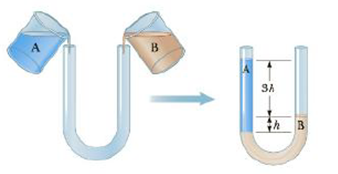Chapter 9, Problem 5CQ

Chapter
Section
Textbook Problem

Equal volumes of two fluids are added to the U-shaped pipe as shown in Figure CQ9.5. The pipe is open at both ends and the fluids come to equilibrium without mixing, (a) Which fluid has the higher density, fluid A or fluid B? (h) What is the ratio ρb/ρA of the fluid densities?Figure CQ9.5

(a)

To determine
The fluid with higher density.

Explanation

The fluid B rises along the column corresponding to A...

(b)

To determine
The ratio of density of B and density of A.

Still sussing out bartleby?

Check out a sample textbook solution.

See a sample solution

The Solution to Your Study Problems

Bartleby provides explanations to thousands of textbook problems written by our experts, many with advanced degrees!

Get Started

Name the following compounds. a CaO b Mn2O3 c NH4HCO3 d Cu(NO3)2

General Chemistry - Standalone book (MindTap Course List)

Describe diffusion in terms or entropy.

Biology: The Unity and Diversity of Life (MindTap Course List)

What is the difference between type I and type II supernovae?

Horizons: Exploring the Universe (MindTap Course List)

Match the term listed in Column A with its definition from Column B

Nutrition Through the Life Cycle (MindTap Course List)